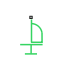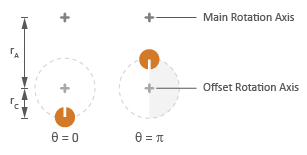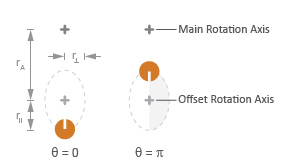•Libraries:
Simscape / Driveline / Inertias & Loads

## Description

The Unbalanced Load block represents the effects of a load whose rotational inertia varies with the rotation angle. Examples include the wobbling of a spinning axle and shaking of an off-center rotating machine. You can specify the load inertia in terms of its rotation path or as an angle-inertia lookup table.

The block provides two rotation path parameterizations. One assumes circular motion of the unbalanced load. The other assumes elliptical motion. In each case, the distance between the unbalanced load and the main rotation axis varies with the rotation angle. The varying distance causes the moment of inertia, given by the parallel-axis theorem, to vary during rotation:

`$I\left(\theta \right)={I}_{0}+M{r}^{2}\left(\theta \right),$`

where:

• I is the moment of inertia about the main rotation axis.

• I0 is the moment of inertia about the intrinsic rotation axis of the unbalanced load.

• M is the total mass of the unbalanced load.

• r is the distance between the unbalanced load and the main rotation axis.

The angle dependence of the moment of inertia leads to an angle dependence of the inertial torque acting on the unbalanced load:

`${T}_{I}\left(\theta \right)=I\left(\theta \right)\stackrel{˙}{\omega },$`

where:

• TI is the inertial torque.

• ω is the angular velocity of the load.

If the load moves in a circular path, its distance to the main rotation axis is given by

`$r\left(\theta \right)=\sqrt{{\left({r}_{C}cos\left(\theta \right)+{r}_{A}\right)}^{2}+{\left({r}_{C}sin\left(\theta \right)\right)}^{2}},$`

where:

• rA is the distance between the main rotation axis and the offset rotation axis.

• rC is the radius of the circular path.

Circular Rotation PathIf the load moves in an elliptical path, its distance to the main rotation axis is given by

`$r\left(\theta \right)=\sqrt{{\left({r}_{\parallel }cos\left(\theta \right)+{r}_{A}\right)}^{2}+{\left({r}_{\perp }sin\left(\theta \right)\right)}^{2}},$`

where:

• r is the radius of the elliptical path in line with the distance from the main rotation axis.

• r is radius of the elliptical path perpendicular to the distance from the main rotation axis.

Elliptical Rotation Path## Ports

### Conserving

expand all

Mechanical rotational conserving port associated with the shaft connection to the unbalanced load.

## Parameters

expand all

Choice of parameterization for modeling the moment of inertia of the unbalanced load. Select `Circular rotation` or `Elliptical rotation` to parameterize the load inertia in terms of the load’s rotation path. Select ```By table lookup``` to provide the load inertia directly across its rotation path.

Constant moment of inertia about the intrinsic rotation axis of the unbalanced load.

#### Dependencies

To enable this parameter, set Parameterization to `Circular rotation`.

#### Dependencies

To enable this parameter, set Parameterization to `Circular rotation`.

Distance between the main rotation axis and the center of the rotation path.

#### Dependencies

To enable this parameter, set Parameterization to `Circular rotation`.

#### Dependencies

To enable this parameter, set Parameterization to `Circular rotation`.

Length of the semimajor axis of the elliptical rotation path traversed by the unbalanced load. The semimajor axis is in line with the line segment joining the main rotation axis and the elliptical rotation center.

#### Dependencies

To enable this parameter, set Parameterization to `Elliptical rotation`.

Length of the semiminor axis of the elliptical rotation path traversed by the unbalanced load. The semiminor axis is perpendicular to the line segment joining the main rotation axis and the elliptical rotation center.

#### Dependencies

To enable this parameter, set Parameterization to `Elliptical rotation`.

Array of angular positions at which to specify the load inertia. The block calculates the load inertia between the angles specified using linear interpolation.

#### Dependencies

To enable this parameter, set Parameterization to `By table lookup`.

Array of load inertias about the intrinsic rotation axis of the load. Each inertia corresponds to an angle specified in the Angle vector parameter.

#### Dependencies

To enable this parameter, set Parameterization to `By table lookup`.

## Version History

Introduced in R2015b